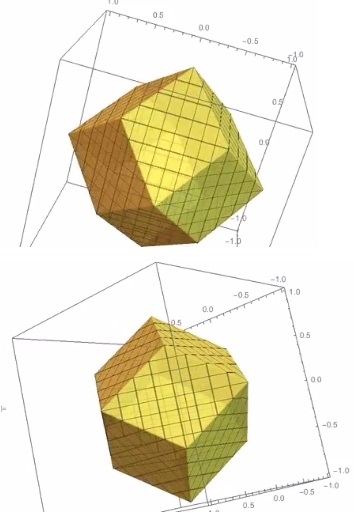# Problem CC156 From Crux Mathematicorum

### Problem### Solution

If the feasible region is constructed in the first octant, the feasible region can be extended to the other octants using symmetry (flipping the signs of $x$, $y$, and $z$, one at a time).

Consider the sides of the planes $x+y=1$, $y+z=1$, and $z+x=1$ that contain the origin. The intersection of the three regions is the feasible region in the first octant.

Any two of these three planes intersect in a line. For example, the planes $x+y=1$ and $y+z=1$ intersect in the line with $x=z$ and $y=1-z$. The remaining two combinations can be written down by cyclically permuting the variables.

The only point where all the three planes and thus the three lines meet is $(1/2,1/2,1/2)$.In the figure, O:$(0,0,0)$, A:$(1,0,0)$, B:$(0,1,0)$, C:$(0,0,1)$, P:$(1/2,1/2,1/2).$ $PAB,$ $PBC,$ $PCA$ are the planes $x+y=1,$ $y+z=1,$ $z+x=1,$ respectively. The common point is $P$. The line segments associated with the plane intersections are also visible.

Using symmetry, we get the feasible region (the outer boundary is shown in the figure below).### Illustration### Acknowledgment

This is problem CC156 (Contest Corner 156) from the Canadian Crux Mathematicorum. The solution is by Amit Itagi, the illustration by N. N. Taleb. A follower Eddie on twitter identified the shape as rhombic dodecahedron.